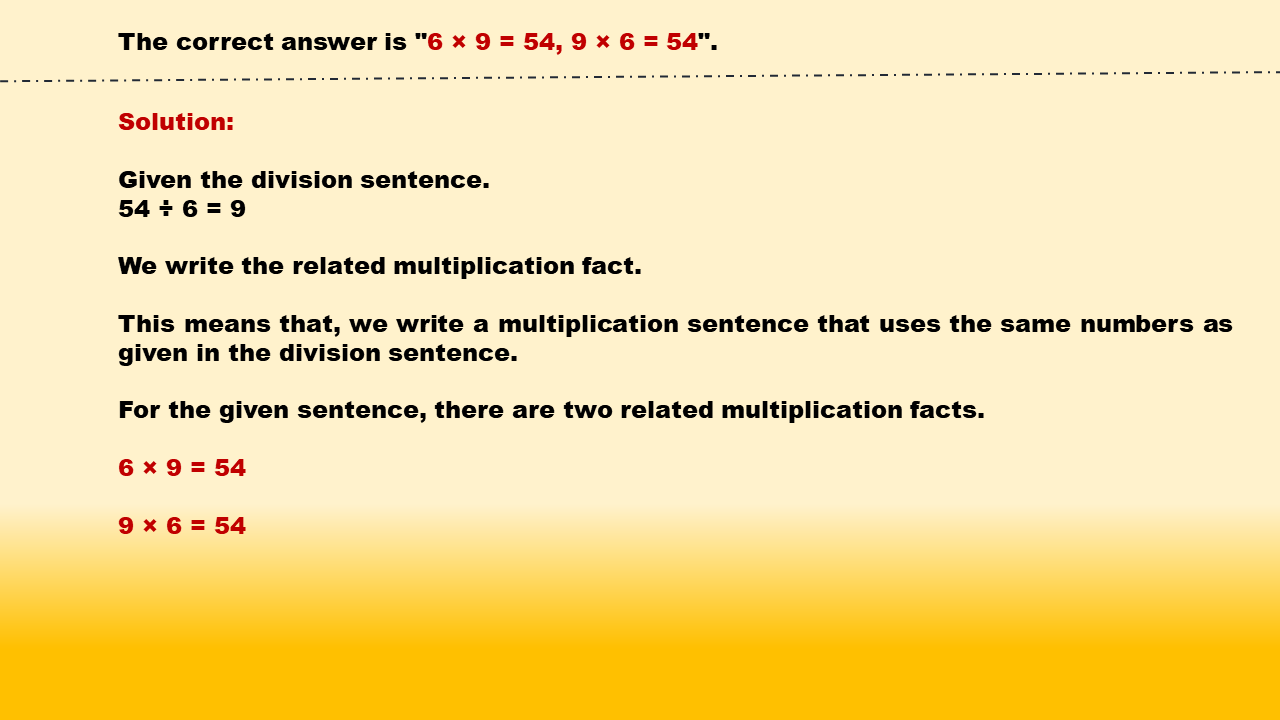1
visibility

Identify related multiplication facts for 54 ÷ 6 = 9.

• 6 × 2 = 12, 2 × 6 = 12

• 6 × 7 = 42, 7 × 6 = 42

• 6 × 9 = 54, 9 × 6 = 54

• 6 × 4 = 24, 4 × 6 = 24